American Journal of Software Engineering and Applications
Volume 5, Issue 6, December 2016, Pages: 40-45

Development of Matlab-Based Software for Peak Load Estimation and Forecasting: A Case Study of Faculty of Engineering, Imo State University Owerri, Imo State, Nigeria

Idorenyin Markson1, Obinwa Christian Amaefule2, Umoren Ifiok Anthony3

1Department of Mechanical Engineering, University of Uyo, Uyo, Nigeria

2Department of Electrical Engineering, Imo State University (IMSU), Owerri, Nigeria

3Department of Electrical/Electronic and Computer Engineering, University of Uyo, Uyo, Nigeria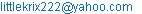(O. C. Amaefule)

Idorenyin Markson, Obinwa Christian Amaefule, Umoren Ifiok Anthony. Development of Matlab-Based Software for Peak Load Estimation and Forecasting: A Case Study of Faculty of Engineering, Imo State University Owerri, Imo State, Nigeria. American Journal of Software Engineering and Applications. Vol. 5, No. 6, 2016, pp. 40-45. doi: 10.11648/j.ajsea.20160506.11

Keywords: Peak Load, Estimation, Forecasting, MATLAB, Power Factor, Waterfall Methodology, Logarithmic Model

Contents

1. Introduction

The reliability of any electric power system rests on its ability to deliver the power demanded from it and at good quality of supply. As such, in power system development planning, effort is made to accurately estimate the immediate demand as well as forecast the future load demands for more years ahead. Peak load demand is used in energy demand management to describe a period in which electrical power is expected to be provided for a sustained period at a significantly higher than average supply level. Peak load demand fluctuations may occur on daily, monthly, seasonal and yearly cycles. For most establishments, the actual point of peak demand is a single half hour or hourly period which represents the highest point of customer consumption of electricity in the establishment. Peak load establishes the highest power consumption in a period.

Accurate models for electric power load forecasting are essential to the operation and planning of an establishment. In this paper, MATLAB-based software is developed for peak load estimation and forecasting. A case study of faculty of Engineering in Imo State University (IMSU) Owerri is selected for the data collection and testing of the software. The software has a user-friendly peak load estimation module for collecting and estimating the peak load based on the power rating of electrical appliances in the faculty. Then a versatile load forecasting module is also designed to forecast the future peak load demand based on the present peak load and some developmental factors in the load forecast interval. In all, for any selected forecast period, the software generates the existing peak load estimation and forecasted peak load values in tables and graphs.

2. Review of Related Works

Electrical Load can be defined as the sum of the requirements in kilowatts (kW) of all consumers in an establishment. Electrical load may also refer to the power consumed by a circuit. Electrical load therefore refers to the cumulative sum of all individual load impedances in an electrical establishment. Peak Load is also used interchangeably with peak demand . It describes a period in which electrical power is expected to be provided for a sustained period at a significantly higher than average supply level. Peak load fluctuations may occur on daily, monthly, seasonal and yearly cycles. For most establishments, the actual point of peak demand is a single half hour or hourly period which represents the highest point of customer consumption of electricity in the establishment. Peak load establishes the highest power consumption in a period.

Basically, forecasts are predictions of future events and forecasting is the act of making such predictions [2,3]. Again, load demand forecasting is a systemic process of quantitatively determining future load demand . Hence, load forecasting is the technique for prediction of electrical load . Load forecasting helps an organisation to make important decisions including decisions on purchasing and generating electric power, load switching, and infrastructure development. Generally, load forecasting can be classified into long-term and short-term functions. In any case, some studies include the concept of medium forecasting which runs from a week to a year [5,6,7,8].

The short-term forecast requires knowledge of the load from one hour up to a few days. Short-term load forecasts are vital to the system operations in terms of short-term unit maintenance work, weekly, daily, and hourly load scheduling of generating units, economic and secure operation of power systems [9, 5, 10]. Some of the techniques used for this type of forecasting are multiple regression, exponential smoothing, iterative weighted least squares and stochastic time series [8,11].

Medium forecasts: This is usually from a week to a year.

Long-term forecasts: This is longer than a year.

The forecasts for different time horizons are important for different operations within an organisation. A large variety of mathematical methods have been developed for load forecasting. Accurate models for electric power load forecasting are essential to the operation and planning of a utility company .

In the last few years, several forecasting methods have been developed. Two of the methods; end-user and econometric approach are broadly used for medium- and long-term forecasting. A variety of methods, which include the similar day approach, regression models, time series, neural networks, expert systems, fuzzy logic, and statistical learning algorithms, are used for short-term forecasting . The development, improvements, and investigation of the appropriate mathematical tools will lead to the development of more accurate load forecasting techniques. Statistical approaches usually require a mathematical model that represents load as function of different factors such as time, weather, and customer class . Regression is the one of most widely used statistical techniques. For electrical load forecasting regression methods are usually used to model the relationship of load consumption and other factors such as weather, day type, and customer class [12,13].

Time series methods are based on the assumption that the data have an internal structure, such as autocorrelation, trend, or seasonal variation [14,12] Time series forecasting methods detect and explore such a structure. In particular, ARMA (autoregressive moving average), ARIMA (autoregressive integrated moving average), ARMAX (autoregressive moving average with exogenous variables), and ARIMAX (autoregressive integrated moving average with exogenous variables) are the most often used classical time series methods .

In this paper load forecasting was implemented using logarithmic method. This is because the plot of the available peak load estimate for the case study gives a logarithmic curve with polynomial of the form Yi = a + bXi + c Xi2.

3. Methodology

The case study for the Peak load estimation is the faculty of Engineering at IMSU. First, peak load data are collected for the following years; 2011, 2012, and 2013. After detailed analysis of the peak load estimate, a working peak load forecast model was analytically developed to produce peak load forecast values for years 2014 to 2020. In order to collect and process the raw peak load data and hence carry out the load forecast, a MATLAB-based program was used. The MATLAB-based program was developed in accordance with the Waterfall software development methodology. The Waterfall software development methodology is a sequential development approach in which development is seen as flowing steadily downwards (like a waterfall) through the phases of requirement analysis, design, implementation, testing (validation), integration and maintenance. The MATLAB-based program has two modules:

1.   Peak Load Estimation Module: This module is used to capture and process the peak load data from the raw peak load data collected from the faculty of Engineering at IMSU. After processing the raw data, the Peak Load Estimation Module provide the sum of peak load per equipment (refrigerator, computer system, ceiling fan, etc) and the overall sum of peak load for the entire faculty of Engineering.

2.   Peak Load Forecasting Module: This module is used to forecast the peak load for selected number of years ahead. The module makes use of the analytically developed peak load forecast model and the overall sum of peak load for the entire faculty of Engineering obtained from the Peak Load Input Module. It then generates peak load forecast results which are presented in tables and graphs.

Again, the case study for the project is the Engineering Faculty of at IMSU. The facilities in the Engineering Faculty at Imo State University consist of office buildings, classroom blocks, workshops and laboratories. Each of these facilities has electrical installations and hence the peak load demand of the faculty is determined by collecting the load demand data of each electrical installation in the facilities that belong to the Engineering faculty. The peak load data was collected on a yearly basis based on the available electrical installations in the faculty as at each of the years in the time interval from 2011 to 2013. Total peak load demand for each year was presented originally in Watts. With a power factor of 0.8, conversion was made to Kilo Volt Ampere (kVA) for each year with the relation below;

Power in kVA = cos Ѳ * kW         (1)

where Ѳ is the phase angle and cos Ѳ is the power factor.

Table 3.1 and Figure 3.1 give the summary of peak load demand for the years 2011, 2012 and 2013 in the Engineering Faculty at IMSU.

Table 3.1. Summary of Load Data for 3 years.

 Interval in Years Power factor (cos Ѳ) = 0.8 Year 1 (2011) Year 2 (2012) Year 3 (2013) Peak Load Demand (W) 74363 79975 83075 Peak Load Demand (kW) 74.363 79.975 83.075 *Peak Load Demand (KVA) = cos Ѳ * Kw 59.49 63.98 66.46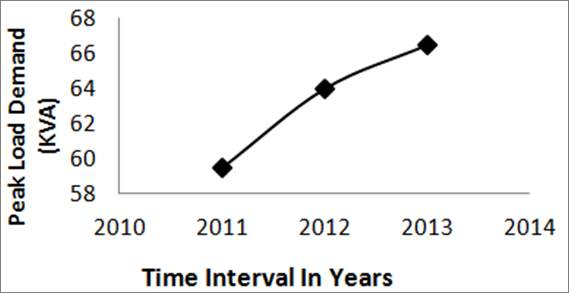Figure 3.1. Peak Load Demand (KVA) vs Time Interval in Years.

3.2. Development of the Peak Load Demand Forecast Model

The peak load forecasting was implemented using logarithmic method. This is because, from Table 3.1 and Figure 3.1, for the period (2011, 2012 and 2013), the peak load demand for the Engineering Faculty at IMSU is a logarithmic curve with the polynomial as shown in Eq. 2a.

Yi = a + bXi + cXi2            (2a)

The error is defined for any point (Xi, Yi) as

ei = Yi – (a + bXi + cXi2)         (2b)

where ei is the error value.

Using method of quadratic regression (least squares polynomial) the sum of squares of errors for n points is given as J where:

J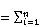(ei.ei)            (3)

Putting Eq 2b into Eq 3 gives:

J(Yi – a – bXi – cXi2) (Yi – a – bXi – cXi2)  (4a)

:. J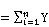2i – 2a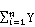i - 2b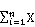iYi – 2ci2Yi + a2n + 2abi + b2i2 + 2aci2 + 2bci3 + c2i4.             (4b)

In order to minimize J, the partial derivatives of J are obtained with respect to a, b, c and equate each of the derivatives to zero, thus

Derivative with respect to a:

j/∂a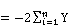i + 2an + 2bi + 2ci2 = 0  (5)

Making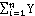i the subject of equation (others divide by two):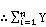i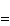an + bi + ci2.       (6)

Derivative with respect to b:

j/∂b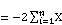iYi + 2a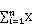i + 2bi2 + 2ci3 = 0 (7)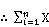iYi = ai + bi2 + ci3  (8)

Also, derivative with respect to c:

j/∂ci2Yi + 2ai2 + 2bi3 + 2ci4. (9)i2Yi = ai2 + bi3 + ci4.  (10)

Table 3.2. The Values Of The Variables Derived From Equations 6, 8 And 10.

 Xi (year) Yi (kVA) Xi2 Xi3 Xi4 Xi Yi Xi2Yi 1 (2011) 59.49 1 1 1 59.49 59.49 2 (2012) 62.99 4 8 16 126 252 3 (2013) 66.46 9 27 81 199.4 598.1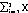i = 6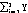i = 188.94i2 = 14i3 = 36i4 = 98iYi = 384.85i2Yi = 909.59

Equations 6, 8 and 10 are simultaneous equations with a, b, c as constants, Xi is independent variable and Yi is dependent variable. The values of a, b, c are calculated using Xi and Yi as shown in Table 3.1, where Yi is the peak load demand, Xi is the corresponding year and n is the number of years which is 3. Table 3.2 shows the values of the variables derived from equations 6, 8 and 10.

Equations 6, 8 and 10 can now be written as simultaneous linear equations (where number of years, n = 3):

3a + 6b + 14c = 188.94           (11)

6a + 14b + 36c =384.85           (12)

14a + 36b + 98c = 909.59          (13)

Equations 11, 12 and 13 can be expressed in matrix form as follows: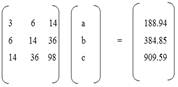(14)

Using Gaussian elimination method, Eq 14 can be solved for a, b and c to give

a = 55.96; b = 3.545; c = - 0.015

Substituting the values of a, b and c into Eq 2a gives the general equation of the peak load demand curve, as

Yi = 55.96 + 3.545 Xi - 0.015 Xi2     (15)

Eq15 represents the peak load demand (power consumption) model and can be used for analysis of the future peak load demand. It is known as the load demand forecast function.

Having obtained the peak load demand function, the future peak load demand can now be calculated by substituting x = 1, 2, 3, … 10 into Eq. 15. Specifically, the peak load demands are obtained for years 2011, 2012, 2013, …, 2020. Therefore, in 2014, Xi = 4.

Y = Y4 = 55.96 + (3.545 x 4) - (0.015 x 42) = 69.9 kVA(69.9)

Similarly, the following applies for subsequent years, however the MATLAB-based software auto-generates the values through the formula relation.

Y = Y5; Y = Y6; Y = Y7; Y = Y8; Y = Y9; and Y = Y10.(10.)

4. Results and Discussions

4.1. The Peak Load Estimation Module

The Peak Load Estimation Module for the peak load estimation comprises of both input interface and output interface used to capture peak load estimation input data and to display the processed peak load estimation data. The input interface is used to collate the number (quantity) and load demand (power rating) of each category of the electrical appliance Figure 4.1.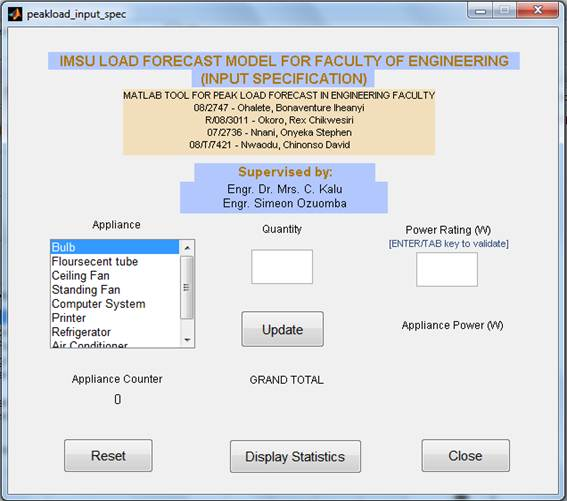Figure 4.1. Screenshot Of The Input Interface Unit Of The Peak Load Estimation Module.

The Output Interface displays a statistical summary of the peak load demand of each category of the appliances included in the estimation data, along with the overall sum of peak load demand of all the appliances listed in the estimation data, Figure 4.2.Figure 4.2. Screenshot of The Output Interface Unit of The Peak Load Estimation Module.

4.2. The Peak Load Forecasting Module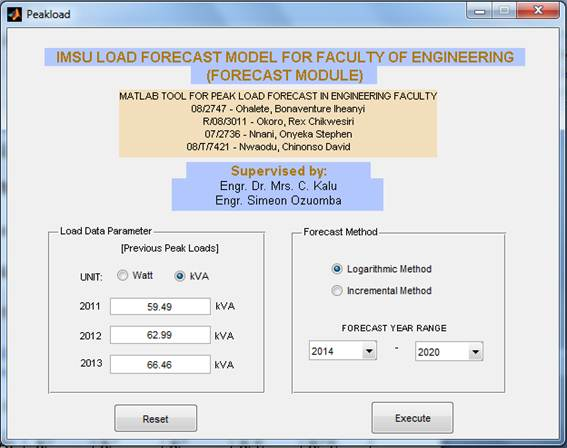Figure 4.3. Screenshot of The Input Unit of The Load Forecasting Module.

The peak load forecasting module has three interfaces; Input Unit, Preview Unit and Output Unit. The input unit of the peak load forecasting module comprises of the Peak Load Data Parameters, Load Forecast Method and Load forecast interval in Years, Figure 4.3. The input data (Figure 4.3 ) for the analysis was done with year 2011 having a load of 59.49kV, year 2012 with 62.99kV, and year 2013 with 66.46kV, where the data is obtained from Tables 3.1 and Figure 3.1.

In order to prevent incorrect data supply, the software was designed to perform input data validation. Specifically, the software will prompt an error dialog box under the scenarios presented in Table 4.1.

Table 4.1. Error Prompts for Input Validation.

 Error Prompt Trigger Event One or more field(s) empty At least one field is not filled Input must be a number Alphanumeric data or special character was supplied in any of the fields in Load Data Parameter Invalid Year Range The ‘from’ Year value is greater than the ‘to’ Year value such as 2019 – 2015

The preview unit of the peak load forecasting module, Figure 4.4 renders supplied data from the input phase with no editable field on its own. It provides the user option to either proceed with forecast computation or return to input interface for correction. It also provides documentation link for the selected method, thereby allowing the user to view the details about the peak load forecast model that is being used for the load forecast.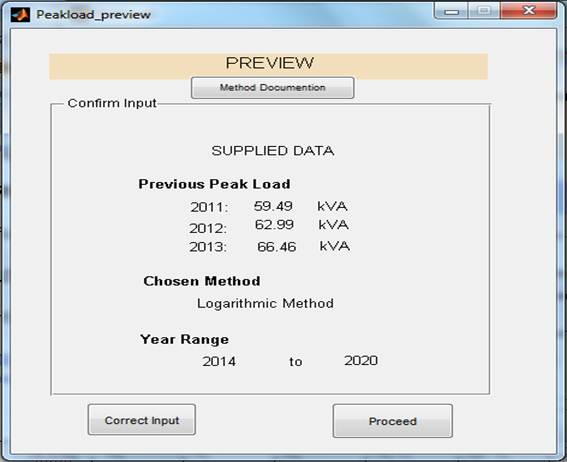Figure 4.4. Screenshot Of The Preview Unit Of The Load Forecasting Module.

The output unit of the peak load forecasting module consists of two output subunits; The Result Tabulation Subunit and The Graph Subunit. In the Result Tabulation Subunit, the peak load forecast result are computed and displayed in a tabular form, Figure 4.5. It only displays results for the selected range of years for the load forecast; in this case from, 2014 to 2020.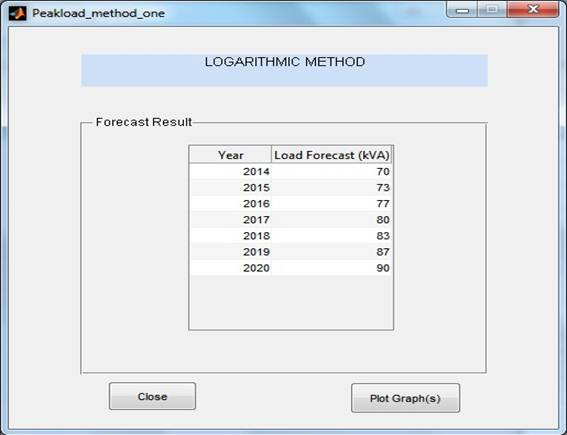Figure 4.5. Load Forecasting Module – The Result Tabulation Subuni.

The Graph Subunit automatically plots the graph of the forecast results, Figure 4.6. Although data values for 2011, 2012 and 2013 are not included in the output table in Figure 4.5, the software fetches their values from the input data in Figure 4.3 and then plots the required graph from 2011 to 2020. The Graph Subunit also provides an option to return back to input interface in case the user has another set of data to forecast.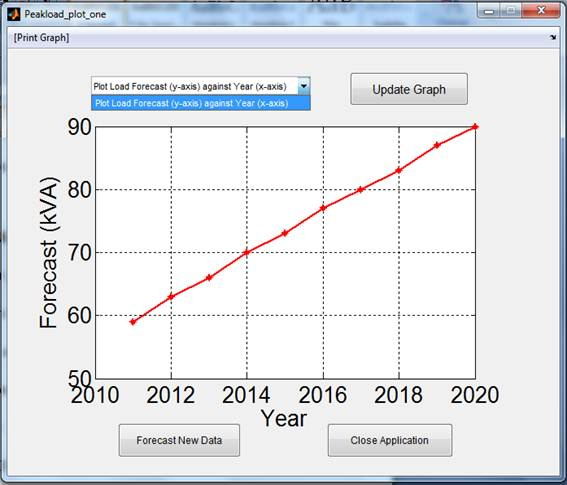Figure 4.6. Load Forecasting Module – Graph Subunit.

4.3. Discussion of Results

The peak load forecast results are presented in two ways; Result Data Tabulation in Fig 4.5 and Graph Relation in Fig 4.6. The load estimates as seen in the result tabulation of Fig 4.5 and graph of Fig 4.6 is a function of the three-year peak load estimate supplied as input data in Fig 4.3; the three-year peak load estimates are actually obtained from Table 3.1 or Fig 3.1. In this case, the three-year input data are for years 2011, 2012 and 2013. The forecast years are year 2014 through to year 2020. The graph plots in Fig 4.6 shows that the load increases as the years go from 2014 through to year 2020. The forecast indicated is therefore realistic on the following basis:

1.   The present growth rate is maintained in the faculty i.e. rate of procurement, building projects executed, etc. The faculty is a young faculty as such new building and other facilities are steadily added to the faculties year after year. This trend occurred in the three years, namely 2011, 2012 and 2013 from which the input data were obtained.

2.  In addition to the addition of new facilities, the existing gadgets (refrigerators, air conditioners, etc) are maintained or replaced with new ones.

Consequently, the forecast indicates that by the year 2020, the faculty of Engineering in IMSU will require about 90 KVA of peak load.

5. Conclusion and Recommendation

5.1. Conclusion

An electric power system can be said to be reliable when it delivers the power demanded from it and at good quality without breaking down in most cases due to overloading. This project therefore addressed the issue of proper load estimation and forecasting, with the advantage of knowing the power supply rating needed at any given time in an organization in this case, the Faculty of Engineering of Imo State University Owerri. The software approach therefore presents an advantage as it provides faster analysis coupled with the graphical result presentations. With this program, important decisions such as power equipment purchase, infrastructure development, yearly and power budget estimations will be made with ease and with greater accuracy.

5.2. Recommendation

Presently, the software supports yearly data input and forecast. Further work is required to make the software to allow user to choose duration of load forecast such as weekly, monthly, quarterly, etc.

Further work is also required to make the software to allow more peak load forecast methods to be available and selectable to users rather than making users to stick to the only option, the logarithmic method used in this paper.

References

1. Saman, W., & Halawa, E. (2009). NATHERS–Peak Load Performance Module Research.
2. Amlabu C. A., Agber J.U., Onah C. O., Mohammed S. Y. (2013) Electric Load Forecasting: A Case Study of the Nigerian Power Sector. International Journal of Engineering and Innovative Technology (IJEIT) Volume 2, Issue 10, April 2013
3. Bower man B.L., O‟Connell R.T, and Koehler A.B. (2005) Forecasting, Time Series, and Regression: An Applied Approach," 4th ed. California: Thomas Brooks/ Cole, 2005.
4. S.S. Vadhera, Power system Analysis and stability, Khanna Publishers Delhi, 2004.
5. Sheikh S. K., Unde M. G. (2012) Short-Term Load Forecasting Using Ann Technique. International Journal of Engineering Sciences & Emerging Technologies, Volume 1, Issue 2, pp: 97-107 ©IJESET
6. S.S. Vadhera, Power system Analysis and stability, Khanna Publishers Delhi, 2004.
7. J.H.Chow, F.F.Wu, J.A.Momoh, Applied Mathematics for restructured electric power systems,  J. Campillo, F. Wallin, D. Torstensson, I. Vassileva. Energy Demand Model Design For Forecasting Electricity Consumption And Simulating Demand Response Scenarios In Sweden. International Conference On Applied Energy Icae 2012, Jul 5--8, 2012, Suzhou, China Paper Id: Icae2012-- A10599
8. IEEE Transactions on Power Systems, Vol. 8, No. 1, February 1993.
9. G. Bharadwaj Aarti And K. Meeta, Demand Forecasting For Electricity: The Indian experience. 2001, pp. 175--192.
10.  H. Alfares And M. Nazeeruddin, "Electric Load orecasting: Literature Survey and classification of methods," International Journal Of Systems Science, vol. 33, no. 1, pp. 23-34, 2002.
11. Feinberg, E. A., & Genethliou, D. (2005). Load forecasting. In Applied mathematics for restructured electric power systems (pp. 269-285). Springer US.
12. Engle R.F., Mustafa C., and Rice J.. (1992) Modeling Peak ElectricityDemand. Journal of Forecasting, 11:241–251, 1992.
13. Charytoniuk W., Chen M.S., and Van Olinda P. (1998) NonparametricRegression Based Short-Term Load Forecasting. IEEE Transactionson Power Systems, 13:725–730, 1998.
14. Chukwukelue, O. P., Daniel, E. C., Chuka, C. E., & Sunday, A. P. (2013) Time Series and Statistical Analysis of Plastic Yield: A Case Study of Finoplastika Industries Ltd, Nigeria. International Journal Of Multidisciplinary Sciences And Engineering, Vol. 4, No. 2, February 2013

 Contents 1. 2. 3. 3.1. 3.2. 4. 4.1. 4.2. 4.3. 5. 5.1. 5.2.
Article ToolsAbstractPDF(384K)